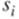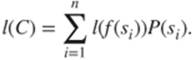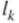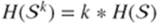﻿ ﻿Information Theory - Information Theory and Compression - Foundations of Coding: Compression, Encryption, Error Correction (2015)

# Foundations of Coding: Compression, Encryption, Error Correction (2015)

### Chapter 2. Information Theory and Compression

The previous chapter already contains several coding principles for compression: fax coding, instantaneous coding, the principle of entropy, and so on. We now carry out a deeper and more exhaustive survey of these principles, from the understanding of why compression is theoretically possible to examples of compression codes which are commonly used in computer science and in everyday life for storing and exchanging music and videos.

The objective is to improve the transmission time of each message as well as the storage space. For this purpose, one has to build codes that optimize the size of messages.

Here we assume that the channel is not subject to perturbations (one says that encoding is without noise); error handling will be studied in the last chapter. We are going to build encoding techniques, which enable one to choose efficient codes, as well as an important theory in order to quantify the information included in a message, to compute the minimum size of an encoding scheme and thus to determine the “ value” of a given code.

We first focus on lossless data compression, that is, compression followed by decompression does not modify the original file. The first section describes the theoretical limits of lossless compression, and the second algorithms to approach those limits. Then the third section shows how changes of representations can expand these presupposed boundaries, with applications to usual codes.

Then, at the end of this chapter, we introduce several techniques for the compression of images or sounds that allow some loss on the visual or the audio quality by modeling human perception.

Exercise 2.1 (It is Impossible to Compress ALL Files Without Loss)

1. How many distinct files of size exactly N bits are there?

2. How many distinct files of size strictly lower than N bits are there?

3. Conclude on the existence of a method that will reduce the size of any file.

Solution on page 295.

Exercise 2.2 (On The Scarcity of Files Compressible Without Loss)

Show that less than one N-bits file over a million, withis compressible by more than 20 bits, without loss of information. Solution on page 295.

### 2.1 Information Theory

Information theory gives the mathematical framework for compression codes. Recall that an alphabet is a finite set on which messages are composed, containing the information to encode or the information already encoded. The set of letters of the source message (data compression is often called source coding) is the source alphabet, and the set of code letters is the code alphabet. For example, the Latin alphabet is the set of letters we are using to write this text, andis the alphabet used to write the messages that are transmitted through most of the numerical channels.

The set of finite strings over some alphabet V is denoted by, and the image of the source alphabet through the encoding function is a subset ofcalled the set of codewords, or sometimes also simply called the code, especially in information theory.

Therefore, a code C over some alphabet V is a finite subset of. The code is composed of the basic elements from which messages are built. An element m in C is called a codeword. Its length is denoted by. The arity of the code is the cardinal number of V. A code of arity 2 is said to be binary.

For example,is a binary code of arity 2, over the alphabet.

2.1.1 Average Length of a Code

In all this section, for the sake of simplicity and because of their practical importance in telecommunications, we mainly focus on binary codes. Nevertheless, most of the following results can be applied to any code.

As all codewords are not always of the same size, one uses a measure dependent on the frequencies of appearance in order to evaluate the length of the messages that will encode the source. One recalls that a source of information is composed of an alphabet S and a probability distributionover S. For a symbolin a sourceis the probability ofoccurrence of.

Letwith, and let C be a code in, whose encoding function is f (C is the image of S through f). The average length of the code C isExample 2.3.

If, the average length of the scheme is 2.

If, then the average length of the scheme is.

One uses the average length of an encoding scheme in order to measure its efficiency.

2.1.2 Entropy as a Measure of the Amount of Information

We are reaching the fundamental notions of information theory. Let us consider a source. One only knows the probability distribution of this source, and one wishes to measure quantitatively his/her ignorance concerning the behavior of. For instance, this uncertainty is higher if the number of symbols in S is large. It is low if the probability of occurrence of a symbol is close to 1, and it reaches its highest value if the distribution is uniform.

One uses the entropy of a source in order to measure the average amount of information issued by this source.

For example, let us imagine a fair die whose value is only given by comparison with a number we are able to choose: how many questions are required in order to determine the value of the die? If one proceeds by dichotomy, it only takesquestions. Now let us suppose that the die is unfair: one has a probability 1 over 2 and each of the five other values has a probability 1 over 10. If the first question is “ is it 1?” then in half of the cases, this question is enough to determine the value of the die. For the other half, it will require three additional questions. Hence, the average number of questions required is.

Actually, it is still possible to refine this result by noticing that three questions are not always required in order to determine the right value among: if dichotomy splits these five possibilities into two groupsand, then only two additional questions will be required in order to find 2 or 3. Only 5 and 6 will require three questions to be separated. For a large number of draws, it is still possible to improve this method if the questions do not always split the set the same way, for example, inand, so that two questions are alternatively required and so on. By extending this reasoning, one shows that the average number of questions required for the five possibilities is equal to.

Hence, the amount of information included in this throw of die (which can be easily applied generally to any source) is defined intuitively by the average number of questions.

Formally, entropy is defined, for a source, asThis is a measure of the uncertainty relying on a probability law, which is always illustrated using the example of the die: one considers the random variable (source) generated by the throw of an n-face die. We have seen that there is more uncertainty in the result of this experiment if the die is fair than if the die is unfair. This can be written in the following way: for all, according to Property 1 in Chapter 1.

2.1.3 Shannon's Theorem

This fundamental theorem of information theory is known as Shannon's theorem or the theorem of noiseless encoding.

First of all, we formulate the theorem when considering a source without memory.

20

Theorem 20

Letbe a source without memory of entropy. Any code uniquely decodable ofover an alphabet V of size q (i.e.,), and an average length l, satisfiesMoreover, there exists a code uniquely decodable ofover an alphabet of size q, and an average length l, that satisfiesProof

First part: Letbe a code of, uniquely decodable, over some alphabet of size q, and letbe the lengths of the words in C. If, thenfrom Kraft's theorem (see page 69). Letbe such thatfor all. One hasfor all i, and, thusis a probability distribution. Gibbs' lemma (see page 17) can be applied, and one obtainsin other words,Yet, because, one has; Hence, the result.

Second part: Let. As(indeed,), there exists a code ofover an alphabet of size q, uniquely decodable, with lengths of codewords equal to. Its average length is. Then, the property of the ceiling function gives usand, as a consequence,This can be written as. This proves the theorem.

One deduces the theorem for the kth extension of:

21

Theorem 21

Letbe a source without memory of entropy. Any uniquely decodable code inover some alphabet of size q, and an average length, satisfiesMoreover, there exists a uniquely decodable code inover some alphabet of size q, and an average length, that satisfiesProof

The proof of the theorem is immediate: according to Property 2 on page 21, one has.

For any stationary source, the theorem can be formulated as follows:

22

Theorem 22

For any stationary sourceof entropy, there exists some uniquely decodable encoding process over an alphabet of size q, and an average length l, as close as one wants to its lower bound:In theory, it is then possible to find a code endlessly approaching this bound (which depends on the entropy of the source). However, in practice, if the process consists of encoding the words of an extension of the source, one is obviously limited by the number of these words (, which might represent a large number of words). In the sequel, we see several encoding processes as well as their relation with this theoretical bound.

﻿